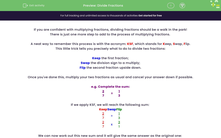# Divide Fractions

In this worksheet, students will practise dividing two fractions by applying the KSF (Keep, Swap, Flip) process and simplifying answers if possible.Key stage:  KS 4

Year:  GCSE

GCSE Subjects:   Maths

GCSE Boards:   Pearson Edexcel, OCR, Eduqas, AQA,

Curriculum topic:   Number, Fractions, Decimals and Percentages

Curriculum subtopic:   Structure and Calculation Fractions

Difficulty level:#### Worksheet Overview

If you are confident with multiplying fractions, dividing fractions should be a walk in the park!

There is just one more step to add to the process of multiplying fractions.

A neat way to remember this process is with the acronym: KSF, which stands for Keep, Swap, Flip.

This little trick tells you precisely what to do to divide two fractions:

Keep the first fraction;

Swap the division sign to a multiply;

Flip the second fraction upside down.

Once you've done this, multiply your two fractions as usual and cancel your answer down if possible.

e.g. Complete the sum:

 2 7
÷
 1 3

If we apply KSF, we will reach the following sum:

Keep Swap Flip
 2 7
÷
 1 3
 2 7
x
 3 1

We can now work out this new sum and it will give the same answer as the original one:

 2 7
x
 3 1
=
 6 7

Clever right?

In this activity, you will practise dividing two fractions by applying the KSF (Keep, Swap, Flip) process and simplifying your answer if you can.

Want a bit more help with this before you begin? Why not watch this short video?

### What is EdPlace?

We're your National Curriculum aligned online education content provider helping each child succeed in English, maths and science from year 1 to GCSE. With an EdPlace account you’ll be able to track and measure progress, helping each child achieve their best. We build confidence and attainment by personalising each child’s learning at a level that suits them.

Get started